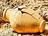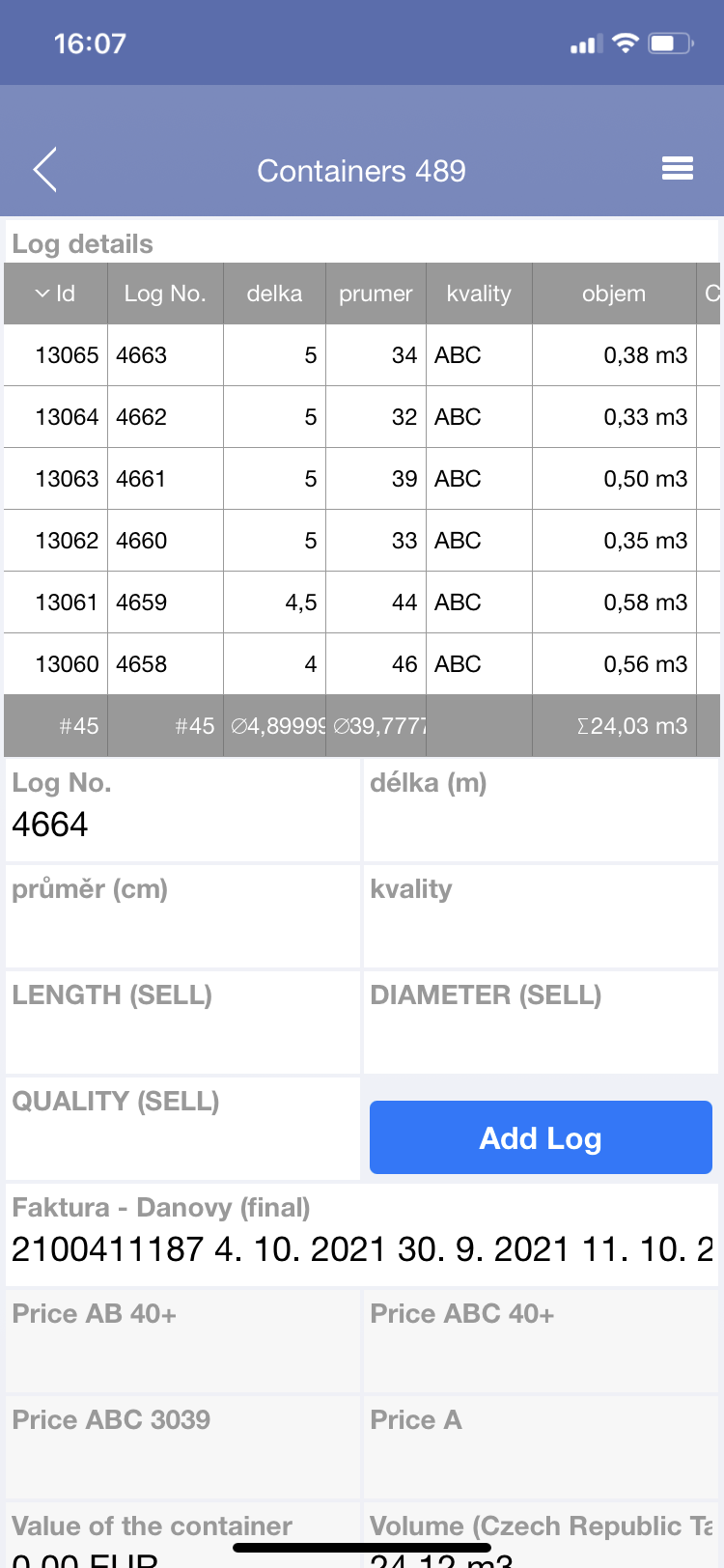### Lagging while creating new records by buttonDear,

I use a botton to create new records like in the code below, it works well for some first attemps, but then some new records dont appear, and we have to close the app on the phone and then re-open to see the records. I installed the app on my iphone 11, and the problem happens in all the other phones our team uses. please advise what we can do. Thank you very much.

let x := Id;
let ln := 'Log No.';
let lb := 'délka (m)';
let db := 'průměr (cm)';
let qb := kvality;
let l := 'LENGTH (SELL)';
let d := 'DIAMETER (SELL)';
let q := 'QUALITY (SELL)';
let i := (create 'Log details');
i.('Log No.' := ln);
i.('delka (koupit)' := lb);
i.('prumer (koupit)' := db);
i.('kvality (koupit)' := qb);
i.('Dia (sell)' := d);
i.('Length (sell)' := l);
i.('Quality (sell)' := q);
i.(Containers := x);
let lnn := number(last('Log details'.'Log No.'));
'Log No.' := text(lnn + 1);
'délka (m)' := null;
'průměr (cm)' := null;
kvality := null;
'LENGTH (SELL)' := null;
'DIAMETER (SELL)' := null;
'QUALITY (SELL)' := null